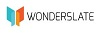# CHEMICAL REACTION ENGINEERING

170.00

 Authors Name K. A. Gavhane Edition First Publishing Year Jun-19 Pages 178 ISBN 9789389108071 Language English Buy E-Book  (PDF Version)[For IOS , Android & Desktop - MCQ Generator Included]

SKU: N4548

For E-Books purchased from Kopykitab.com

For E-Books purchased from Amazon

## Syllabus …

1. ### Kinetics of Homogeneous Reactions (Hrs. 10, M. 10)

1.1   Scope of chemical reaction engineering.

1.2   Variables affecting the rate of reaction.

1.3   Reaction rate, Rate of reaction in various forms, Rate equation, Rate constant, Units of rate constant, Concentration dependent term of rate equation.

1.4   Types of reactions :

(a)  Homogeneous and Heterogeneous reactions.

(b)  Single and Multiple reactions.

(c)  Elementary and non-elementary reactions.

(d)  Molecularity and order of reaction.

(e)  Chain and non-chain reactions.

(f)   Types of intermediates formed in non-elementary reactions.

1.5   Activation energy and its significance.

1.6   Temperature dependent term of rate equation.

Temperature dependency of rate constant from :

(a)  Arrhenius law.

(b)  Transition state theory.

(c)  Collision theory.

(d)  Comparison of different theories.

1. ### Interpretation of batch reactor data (Hrs. 20, M. 20)

2.1   Constant volume batch reactor : Reaction rate.

2.2   Analysis of total pressure data obtained in a constant volume system.

2.3   Concept of fractional conversion xA.

2.4   Methods of analyzing kinetic data, general procedure for integral method of analysis of data, General procedure for differential method of analysis of data, method of isolation, Method of initial rate, Method of least square, Method of excess.

2.5   Integral method of analysis : Integrated rate expression for irreversible unimolecular first order reaction, Irreversible bimolecular second order reaction of the type A + B ® product and 2A ® product, nth order reaction, Zero order reaction, Autocatalytic reactions.

2.6   Parallel and series reactions, Application of half-life method for : Zero order, First order, Second order and nth order irreversible reactions.

2.7   Variable volume batch reactor, Concept of eA, Integral method of analysis based on variable volume system for zero order reaction, First order reaction and second order reaction.

1. ### Introduction to reactor design (Hrs. 14, M. 16)

3.1   Factors to be considered while designing a reactor, Material balance equation for a reactor.

3.2   Types of reactors : Batch reactor, Semi-batch reactor, Continuous stirred tank reactor, Plug flow reactor, Applications of different reactors.

3.3   Relation between CA and XA for constant density and changing density systems at constant temperature and pressure for batch system.

3.4   Performance equation for ideal batch reactor (constant and variable volume system).

3.5   Concept of space time and space velocity.

3.6   Performance equation for steady-state (MFR) Mixed Flow Reactor (constant and variable volume system).

3.7   Performance equation for steady-state (PFR) Plug Flow Reactor (constant and variable volume system), Holding time and space time for flow reactors.

1. ### Design for single reactions (Hrs. 16, M. 16)

4.1   Size comparison of single reactors : Batch reactor v/s Plug Flow Reactor, Multiple Flow Reactor v/s Plug Flow Reactor.

4.2   Multiple reactor systems :

(a)  Plug flow reactors in series and/or in parallel.

(b)  Plug Flow Reactors in parallel-series combination.

(c)  Equal size Multiple Flow Reactors in series.

(d)  Multiple Flow Reactors of different size in series :

(i)     Finding the conversion for a given system.

(ii)    Determining the best system for a given conversion.

4.3   Different types of reactors in series : Best arrangement for set of ideal reactors.

1. ### Catalysis (Hrs. 04, M. 08)

5.1   Nature of catalytic reactions, Important properties of catalyst : Specificity, Activity, Porous structure.

5.2   Promoters, Accelerators and Inhibitors.

5.3   Mechanism of solid catalyzed gas phase reactions.

5.4   Different methods of catalyst preparation : Precipitation, Gel formation, Simple mixing, Impregnation, Types of catalyst poisoning : Diffusion, Deposition, Stability, Selectivity, Sintering, Chemisorbed.

5.5       Methods of catalyst regeneration : Fluidized bed reactor, Packed bed reactor – Concept of hot spot formation in packed bed reactor, Applications of above reactors.